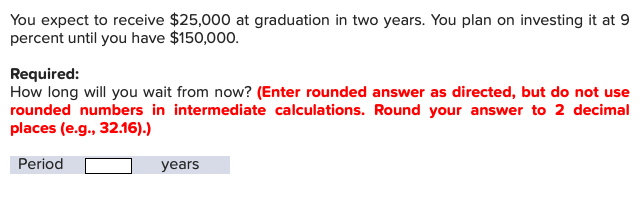You expect to receive \$25,000 at graduation in two years. You plan on investing it at 9percent until you have \$150,000Required:How long will you wait from now? (Enter rounded answer as directed, but do not userounded numbers in intermediate calculations. Round your answer to 2 decimalplaces (e.g., 32.16).)Periodyears

Questionhelp_outlineImage TranscriptioncloseYou expect to receive \$25,000 at graduation in two years. You plan on investing it at 9 percent until you have \$150,000 Required: How long will you wait from now? (Enter rounded answer as directed, but do not use rounded numbers in intermediate calculations. Round your answer to 2 decimal places (e.g., 32.16).) Period years fullscreen
Step 1

Let's say we have to wait for n years once you get the money at graduation, which itself is two years from now.

Sum of money that your will receive at graduation = PV = \$ 25,000

Target future value, FV = \$ 150,000

Annual rate of return, r = 9%

Step 2

So, the connecting equation is:

FV = PV x (1 + r)n

Hence, 150,000 = 25,000 x (1 + 9%)n

Hence, 1.09n = 150,000 / 25,000 = 6

Step 3

Take log to the base "e" on both the sides,

n ln (1.09) = ln (6)

Hence,...

Want to see the full answer?

See Solution

Want to see this answer and more?

Our solutions are written by experts, many with advanced degrees, and available 24/7

See Solution
Tagged in